DANGERS IN MEASURING THE POWER OF AC DEVICES WITH METERS

Patrick Bailey
P.O. Box 201
Los Altos, CA 94023-0201

From: INE/EEMF 2001 Conference
New Energy News (NEN) copyright 2001 by INE.
COPYING NOT ALLOWED without written permission.

Abstract

Simple explanations are given as to why we should not believe the power measured from various alternating current devices when using meters to measure the voltage and the current, and obtaining the power by simply multiplying these values. Simple mathematical analyses confirm that power levels calculated with such "AC meter" measurements can vastly over-predict the real power produced in such devices, and that almost any AC device can be tuned-up and shown to be a "over-unity device" or a "free-energy machine" using such simplistic meter measurements and math. For accurate measurements of both input and output power measurements, a high-bandwidth sweep digital oscilloscope is absolutely necessary to calculate the real power levels. The INE will continue to contact and challenge any such researcher to allow their device to be properly tested, and the results of that challenge and the measurements will continue to be documented in the INE Device Database.

Introduction

The Institute for New Energy (INE) has been formed to examine, research, and summarize any and all the new and advanced energy conversion devices that any inventor and researcher has to offer. The purpose of the INE is to search for and seek out new forms of potential energy than can be converted into usable power, for either heating or for electricity production.

Any device, if found, that can show that it generates more power in the output than it can in the input is called in the literature an "over-unity device" or "a free-energy machine". Such devices and machines are obviously impossible, as energy cannot be created or destroyed.

However B and here is the catch B what if the researcher is not measuring all of the input power; what if some of the input power is stored somewhere as potential energy, and only a small amount of "input energy" is required to somehow convert that potential energy into output energy? If that "inherent potential energy" is not measured in the input power, then the greater output power will cause the device or machine to appear to an "over-unity device" or a "free-energy machine", when we know that it is really not. In reality, the energy is already there, and is just "ready-to-go".

Examples of such "free-energy machines" abound, such as a flashlight, gunpowder, a firecracker, a bullet, any dam, and any nuclear reactor. The energy that is released is much greater than the energy that we put into it, and it thus can appear to be called a free-energy machine - but we know better.

So it is with other forms of potential energy that we have not yet learned to measure, and thus have not discovered or begun to understand. So any device that could harness any such new potential energy, would appear to be a "free-energy machine". Names for some forms of these new un-discovered potential energy sources that researchers have postulated that exist include: Cold Fusion, Low Temperature Nuclear Reactions, Earth Energy, Space Energy, Aether Energy, and Rotational Magnetic Energy. Such devices are being summarized and reviewed in the Institute for New Energy's Device Database . Any device can be entered into this database by completing the device data template for that device .

This paper concerns the measurement of the input and output voltage, current, and power levels of this latter form of postulated potential energy: the potential energy from Rotational Magnetic Energy Devices. The INE website includes many discussions of these other postulated sources of possible potential energy sources.

Rotational Magnetic Energy Devices are those in which coils rotate past magnets, or magnets rotate past coils, in a cyclic manner, to ultimately produce an AC voltage and AC current output. Several devices have been proposed in the literature that are reported to somehow capture some un-discovered potential energy, and thereby achieve an "over-unity" or "free-energy" effect. Some of the devices, most notably, are advertised by Dennis Lee and Better World Technologies. 

It is important to realize that the voltage and current measurements made using ordinary electrical measurement meters, such a lab meters or VTVMs (vacuum tube volt meters) will usually not produce an accurate measurement of the actual average voltage and current present over any given cycle. The meters are usually calibrated to measure a RMS (root-mean-square) value of a pure sinusoidal waveform. Thus an average power CANNOT be calculated by just multiplying meter readings of voltage and current B unless the waveforms are pure sinusoidal without any phase angle between the voltage and the current. This is explained and shown below. Otherwise, almost anyone can sell you their "free-energy machine" that indeed does NOT work.

Such a simple paper as this is absolutely necessary in the open literature for the average person, or possible the average investor, as many people have been "taken" by unscrupulous racketeers that promise such over-unity devices, while providing only deceptions and lies.

Mathematical Preliminaries

The most simple alternating current or voltage waveform (or any waveform for that matter) is a pure sine wave. This is the waveform that you recall from the old TV program "The Outer Limits", and is exactly the waveform that would result when measuring the distance from the center of a circle along any set axis crossing the circle, when a pencil is moved around the circle.

The form of a sine wave is mathematically described as, say for a voltage V (Volts) in an electrical circuit:

V(x) = Vo sin(x)

Where Vo is the maximum voltage, and x varies from 0 degrees to 360 degrees for one complete cycle.

Or, if x is defined in radians, instead of degrees, then x would vary from 0 to 2 Pi radians for one complete cycle, where Pi is the universal constant 3.141592653..., and is the ratio of the circumference of a circle to its diameter:

So:

0 <= x <= 360 Degrees, over [0,360], or equivalently

0 <= x <= 2 Pi Radians, over [0, 2 Pi].

The value of sin(x) goes from 0 at the start of the cycle (x=0 degrees), and goes to its maximum of 1.0 at one-quarter of the cycle (x=90 degrees), goes back down to 0 at one-half of the cycle (x=180 degrees), goes to its minimum of -1.0 at three-quarters of the cycle (x=270 degrees), and finally back to 0 at the end of the cycle (x=360 degrees); and continues in this smooth periodic manner for any revolution, or cycle.

Also, the current A (Amps) in an electrical circuit can also be defined a sine wave of the form:

A(x) = Ao sin(x)

With maximum current amplitude Ao.

Given this voltage and this current, then the power (voltage times the current) would be:

P(x) = Vo sin(x) Ao sin(x) = Vo Ao [sin(x)]**2 = Po [sin(x)]**2

where [sin(x)]**2 means sin(x) times sin(x), or sin(x) squared,

and Po = Vo Ao

So the maximum power varies from 0 to the maximum power Po, which is correctly given as the product as the maximum voltage and the maximum current.

OK. We have no problems with this so far.

Now: Problems occur because:

1. Most voltage and current waveforms are NOT in phase; and

2. Most voltage and current waveforms produced by these rotational machines are not pure sine waves; and

3. Rapid voltage or current spikes will give false readings.

Out of Phase Waveforms

In AC circuits, combinations of resistance, capacitance, and inductance give rise to what is called a "phase angle" that is produced between the voltage and the current waveforms. We shall cause this phase angle d, which is measured like x, either in degrees or radians.

The Phase Angle d.: 0 <= d <= 360 degrees.

The phase angle measures the difference between the start of the voltage waveform from zero and the start of the current waveform from zero during one cycle. For a zero phase angle, the voltage and current are "in phase", and d=0. If the voltage rises before the current, then the voltage is said to be "leading", and the current is "lagging", and visa-versa.

The phase angle is calculated as the angle between the pure resistance in a circuit (measured on the x-axis) and the total reactance of the circuit (measured on the y-axis, with the capactive reactance being measured positive, and the inductive reactance being negative - for the voltage lagging the current). These calculations can be found in any electrical engineering book.

We shall assume here that the voltage is lagging the current (as it really does not matter), for some given phase angle d, and the mathematical equivalent of saying this, for pure sine waves is:

V(x) = Vo sin(x)

A(x) = Ao sin(x+d)

Where 0 <= x <= 360 for one cycle, and 0 <= d <= 180 for any given phase angle.

With the inclusion of this very real phase angle, the real power becomes:

P(x) = Po sin(x) sin(x+d)

Where Po = Vo Ao, as before.

A problem now arises because the maximum voltage occurs at a different time than the maximum current, and separate meter readings will neither account for nor include that!

The effect of this phase angle (d) is seen in Figs. 1 - 4. These figures show a voltage waveform, V(x)=sin(x), and a current waveform, A(x)=sin(x+d), over one cycle, both with a maximum value of 1.0, and also the calculated power as a function of time, P(x). The power also has a possible maximum value of 1.0, when d=0. The effect of increasing the phase angle from 0, to 5, to 30, and to 60 degrees is seen in these figures, as the current is said to "lead" (or start sooner than) the voltage by the phase angle d. Although the voltage and current waveforms remain the same, and are just offset (or moved-over) by this phase angle, the shape of the power waveform radically changes, from 0.5 [sin(x)]**2 for d=0, to more elaborate forms as the phase angle increases. In addition, the power is even seen to become negative when the phase angle becomes just larger than d=4 or 5 degrees.

The interpretation of this "negative power" as seen in these figures can be explained as follows. We have arbitrarily defined the direction of the flow of the electrons (or the holes) in a wire to be "positive" in one direction, and "negative" in the opposite direction. So, if the current is positive, the flow is in that "positive" direction. Thus, if the power is negative, the work capable of being performed is just in the opposite direction, due to either a negative voltage or a negative current, and not both. The effect of this "negative power" is considered below.

Meter Measurements

Meters are usually calibrated so that they measure 0.707 of a sine wave's maximum value. This is called the "RMS" value (root-mean-square value). Here's why:

Let's assume that we want to measure the power in an AC circuit that has the voltage and current as pure sine waves, and in phase. Then, if we measure the voltage and get Vm (for voltage-measured), and measure the current and get Am (for current-measured), then we want the product of those measurements to give us the power measured Pm:

Vm Am = Pm

We also know that the average power for this circuit will be the average of P(x) above for d=0 (in phase) over one cycle.

Without writing the integral equation, the power should be the integral of P(x) over one cycle divided by the length of the cycle. The integral of sin(x)**2 over one cycle is twice the integral of [sin(x)]**2 over one-half a cycle, and 4 times the integral of sin(x)**2 over one-forth of a cycle (due to the symmetry of sin(x) squared), each giving the same answer: 0.5 Po.

So, in order for the meter readings to measure that proper power value, the meter readings must be adjusted (calibrated) to account for that factor, so that:

Pm = Po/2 = 0.5 Po.

We will then measure half of the maximum power with the meter, and so we will get the right answer.

To ensure that, the meter must be adjusted or calibrated at the factory to read only 1/SQRT(2) (or 0.707) of the maximum voltage, or equivalently the maximum current:

Vm = Vo/SQRT(2) = 0.707 Vo.

and

Am = Ao/SQRT(2) = 0.707 Ao.

In short: by calibrating the meter to read only about 71% of the PEAK value of either the voltage or the current, then the product of these meter readings will give a very close approximation to the actual average power B for: (1) pure sinusoidal waveforms and (2) NO or zero voltage-current phase angle.

The meter has now been calibrated.

Now, what happens if we include this real phase angle (that could be anything), or have voltage and current waveforms that are not pure sinusoidal?

In both cases B we can get an apparent and NOT REAL over-unity device.

Effect of Phase Angle

We can assume the power is represented by pure sine waves to examine the effect of phase angle only, by:

P(x) = Po sin(x) sin(x+d)

The average power over the course of one cycle would then be:

Average Power = Integral [ P(x) dx ] / Integral [ dx ]

Where the integral limits would be from 0 to 360 degrees, or 0 to 2 Pi radians.

We know that these indefinite integrals are algebraically equal to [4,5,6]:

Integral [ P(x) dx ] = Po { cos(d) [ x/2 - sin(2x)/4 ] + sin(d) [sin(x) sin(x) /2 ] }

And

Integral [ dx ] = x

So, when evaluating this over one cycle, or [0, 2 Pi] radians, we get exactly and analytically:

Average Power = Po cos(d) /2 = Pm cos(d)

So we see that when the voltage and current are in phase (d=0), the average power is exactly equal to the measured power; ta da!

BUT, when then the phase angle is not zero, the average power is LESS THAN the measured power by an amount equal to the cosine of the phase angle. This term, cos(d), is defined as "the power factor" in most text books. And, all meter readings must be adjusted by multiplying meter-read calculated power Pm by this power factor to get the true integrated or average power.

The effect of the phase angle on the integrated power is seen in Fig. 5, as calculated by a simple digital FORTRAN computer program. Indeed the integrated power follows the equation: Pm cos(d).

Another way of saying the same thing, is that if you can adjust the resistance, capacitive reactance, and inductive reactance of a device so that you get a big phase angle (d) between the voltage and current, and assuming that the voltage and the current are pure sine waves, then the power that you would SEEM TO GET by multiplying the average meter voltage reading and the average current meter reading would be:

Meter Readings Calculated Power = Vm * Am = Average Power / cos(d)

Which would always be greater than the real available average power by the amount 1/cos(d) - which is always greater than 1 for d>0 -

And you might think that this would be an over-unity device, or a free-energy machine!!!

The effect of forgetting the power factor is seen in Fig. 6, where we can plot a "fictitious power gain" that we would get by just multiplying meter readings, when forgetting all about the phase angle and the power factor. It is noted that all we need in our device is enough capactive or inductive reactance at the device's operating frequency to create a phase angle of about 25 degrees - to get a 10% over-unity device!!!

Not!

Effect of "Negative Power" Values

The integration of P(x) dx above is done by calculating the area under the P(x) curve, over one cycle, by varying x from 0 to 2 Pi. This can be thought of as dividing the integral [0,2 Pi] into many divisions, from each x(i) to x(i+1), and calculating the area under P(x) in that interval, which could be approximated using the trapezoidal rule, A(i) = 0.5 [ P(i) + P(i+1)] * [x(i+1) - x(i)]. Then the whole integral would be the sum of all of these little areas.

This integration process then subtracts any negative power values from the total averaged power during that summation. This is equivalent to saying that the useful work that we can get out of this device, the power times some time interval, is measured with respect to one direction. So, any negative work gets subtracted from positive work.

A device could be built to impose this rule, and also it might not. If a device were constructed just right so that any power created in the opposite direction could also be utilized, then the integrated power should include all negative work as positive work. Mathematically, this is saying that we should integrate not the power values, but the absolute value of the power values (regardless of sign).

If we include all of the power generated over one cycle, regardless of sign, we can sum up the absolute values of all those little areas A(i) above in a computer program, and plot the real averaged absolute value of the power, the Avg. ABS Power, as seen in Fig. 5. Since we are summing more area into the integral, we expect the average power per cycle to be greater than the integrated regular power, as is seen.

Of course if you were advertising a fraudulent "free-energy device", you would not want this Avg. ABS Power to be seen in your results, so you would probably design your device to subtract any negative power. Otherwise, your fictitious energy gain would drop to much lower values, as seen in Fig. 6.

Effect of Non-Sinusoidal Waveforms

As meters are calibrated for sinusoidal waveforms, to calculate the appropriate RMS values, the use of non-sinusoidal waveforms will give very unpredictable results. The average power will always depend on the shape of the waveform found by multiplying the voltage and current waveforms. Waveforms with slow changes may produce overly-low meter readings, while waveforms with rapid changes may produce overly-high meter readings.

Effect of Spiked Voltage or Current Waveforms

In many rotating magnetic devices, it is possible to create very rapidly rising voltage and/or current waveforms, such as voltage spikes or square wave pulses. In many cases when using regular meters to measure such spikes, the meter reading will indicate much more voltage or current than is actually present, due to the rapidly increasing signal and the "soft" damping of the needle in the meter. Thus a long series of rapid voltage spikes could cause a meter to read a constant voltage, even though the actual applied voltage is not constant. In this manner a series of voltage spikes and a series of current spikes could cause the average voltage and average current meter readings to appear to be constant, thus appearing to give a constant power when multiplied together, when in actuality the real power could be very small, and even zero if the voltage and current spikes are out-of-phase.

Lighting Lamps to Measure Power

Some people think that if a 100 Watt light bulb can be lit, then there must be 100 Watts flowing in that circuit. This is absolutely NOT TRUE! Just as meter readings can produce false data, so can glowing light bulbs! It can be shown that light bulbs can be illuminated with only a fraction of the electrical power associated with the bulb, by using various pulsed waveforms and either high voltage or high current pulse widths. Again, in these cases, the voltage and current must be digitally measured as a function of time, and the power digitally calculated from those waveforms, to determine the real average power in the circuit.

Recent Investigations

Recently, the "free-energy generator" advertised and promoted by Lou Brits and John Christie located in Cairns, Australia, gained a lot of attention in the US and abroad.  The INE is in contact with a research team that traveled to that city with their own equipment to test that device in the latter part of 2001. Unfortunately, the claims made about this device were found to be false, and its efficiency very high, and not over unity.

The One Watt Challenge

At one of the first Institute for New Energy international conferences, Harold Puthoff submitted a paper entitled the "One Watt Challenge" to be given to any over-unity device researcher; which basically says: "Close the loop and show me you can produce 1 extra Watt of power" - or shut up. This open challenge still stands for all rotating magnetic and AC frequency devices. 

Conclusions

We CANNOT use separate voltage and current meter readings to obtain an average power value in complex AC circuits. These circuits are found in all rotational magnetic devices, generators, and machines.

We MUST INSTEAD use a high sample rate, high bandwidth digital oscilloscope to measure the time-dependent voltage and current waveforms, and to then digitally calculate the power waveform. The average power is then to be calculated from that power waveform over each cycle. If work in both circuit directions can somehow be used in the device, then the absolute value of the power is to be averaged over each cycle.

The only way you can see what the real power is in any circuit in complex AC devices is to:

1. Measure the voltage waveform digitally with a high frequency oscilloscope, and store it over a cycle or multiples of cycles;
2. Measure the current waveform digitally and store it over a cycle or multiples of cycles;
3. Calculate the digital power waveform by digitally multiplying the digital voltage and current waveforms, and
4. Average the power waveform over one cycle, or multiple complete cycles, digitally and carefully.

This can all be easily done with today's high-bandwidth, multi-channel sweep digital oscilloscopes.

The INE will be more than happy to recommend to any researcher the name and address of an independent test engineer who would love to analyze your favorite "free-energy machine" in this manner!!!

Almost all of the so-called "over-unity devices" and "free-energy machines" that you will find advertized in the US and even internationally will be FALSE, because of the facts explained here in this paper.

The INE will continue to track all of the devices and machines that it is aware of, and show any short-comings of each.

The INE will continue to contact all researchers that claim to have such electricity generating machines, and to offer our known research engineers to test and calibrate their device or machine.

All results and ignored requests will be documented and posted on our INE Device Database website.

The INE is continuing to look for the new devices and machines that WILL really work, as new forms of potential energy are continually being discovered and understood.

Remember:

Anytime anyone wants to show you their "over-unity device" or their "free-energy machine" where they are using AC meters to measure the input and out powers , by measuring separate voltage and current readings and then just multiplying them together to get a power reading - run, do not walk, to the door!

References

1. The Institute for New Energy Device Database (Website): http://www.padrak.com/ine/DBGUIDE.html

2. The Institute for New Energy Device Template (Website): http://www.padrak.com/ine/DBTEMPLATE.html

3. United Community Services of America, including info on Dennis Lee's weekly radio show (Website): http://www.ucsofa.com/

4. CRC Standard Math Tables and Formula, 30th Edition, 1996.

5. The Integrator (Website): http://integrals.wolfram.com.

6. S.O.S. Math (Website): http://www.sosmath.com/tables/integral/integ37/integ37.html

7. "World's 1st Commercial Free-Energy Generator", Electrifying Times newspaper, Vol. 8, No. 3, front page article (undated, 2001).

8. "Alternative Energy Sources: Good News/Bad News and the "One watt Challenge", H. E. Puthoff, Proceedings of the International Symposium on New Energy, May 12-15, 1994, Denver, Co, pp. 373-380.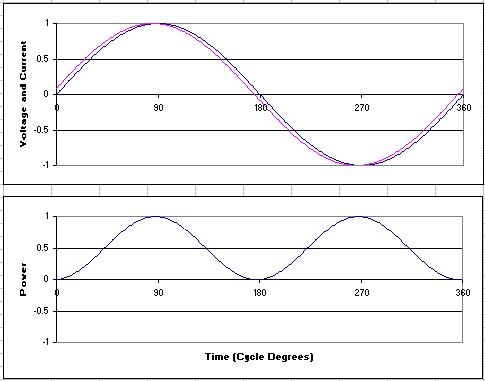Fig. 1: Phase Angle = 5 Degrees. Average Integrated Power = 0.4981.Fig. 2. Phase Angle = 15 Degrees. Average Integrated Power = 0.4830.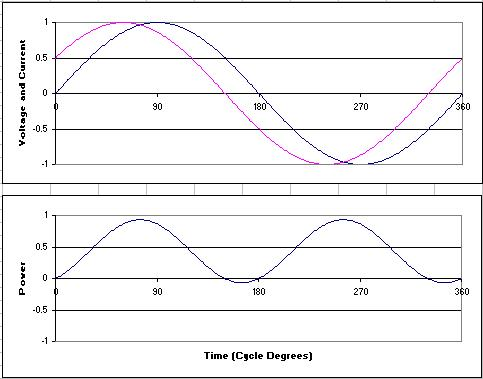Fig. 3. Phase Angle = 30 Degrees. Average Integrated Power = 0.4330.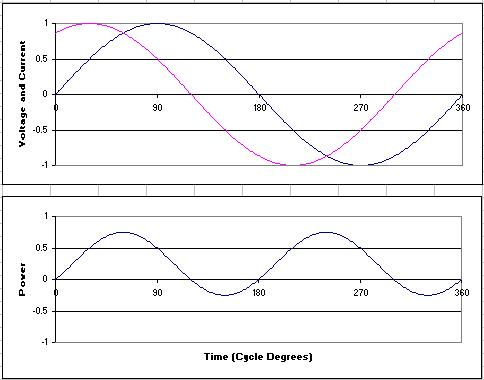Fig. 4. Phase Angle = 60 Degrees. Average Integrated Power = 0.2500.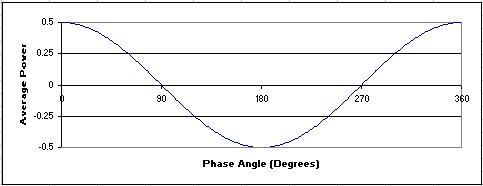Fig. 5. Real Average Integrated Power vs. Phase Angle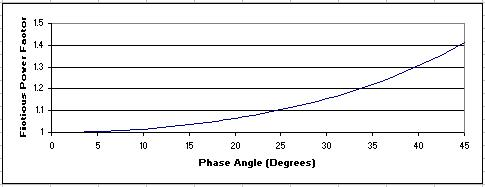Fig. 6. Fictitious Power Increase vs. Phase Angle